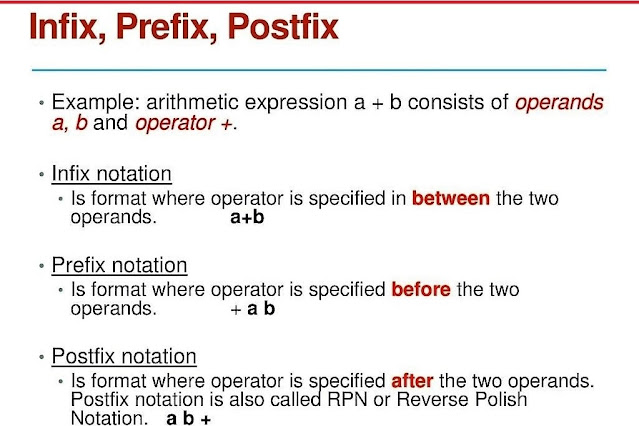# Infix, Prefix and Postfix conversion in C programmingUsing programming, we have to learn how to implement various algorithms. This article will implement infix to prefix, infix to postfix, the prefix to infix, the prefix to postfix, postfix to infix, and postfix to infix calculator using c programming. Let's discuss a bit about infix, prefix, and postfix notation.

#### Infix notation:

Infix is a format of operator placement where the operator is specified between the two operands.

a+b

#### Prefix notation:

In prefix, the operator is specified between the two operands.

+ab

#### Postfix notation:

Postfix notation is where the operator is specified after the two operands. This notation is also called Reverse Polish Notation.

#### Example of postfix notation:

So we can say that the terms infix, prefix, and postfix tell us whether the operator's go-between, before, or after the operands. alert-infoInfix, Prefix, and Postfix conversion in C programming

### Infix, Prefix, and Postfix conversion in C programming

The C program has 5 options to convert:

1. Infix to Prefix
2. Infix to Postfix
3. Prefix to Infix
4. Postfix to Prefix
5. Postfix to InfixMain Menu of Infix, Prefix, and Postfix convertor

### Algorithm for converting an infix expression into postfix operation

1. Add "("at the beginning and ")" at the end of an
infix expression Q.
2. Scan Q from left to right and repeat
Step 3 to step 6.
3  If an operand is encountered, add it into postfix P.
4. If a left parenthesis is encountered,
push it onto the stack S
5. If an operator op is encountered, then,
(a) Repeatedly pop from Stack S and add
it to postfix each operator which has
same precedence as or higher priority than op.
(b) Add op to Stack S.
6. If the right parenthesis is encountered, then
(a) Repeatedly pop from Stack S and add
it to postfix each operator until left parenthesis is encountered on stacks.
(b) Remove the left parenthesis.

#### Algorithm for evaluation of postfix string

1. Scan postfix P from left to right and repeat
Step 2 and 3 for each element of P until the
NULL character or another symbol is encountered.
2. If an operand is encountered, push it onto the Stack.
3. If an operator op is encountered, then
(a) Remove the top elements of stack S where A is the
top element, and B is the next top element
(b) Evaluate B op A
(c) Place the result back onto the stack S
(d) Return the top of the Stack, which is the required result for our calculation.
And finally, the code we use to convert our infix equation to postfix is also able to find the result of the equation.

We will use the Stack in C programming to implement Infix, Prefix, and Postfix, conversion calculators.

### The complete project of infix, prefix, and postfix calculator using C programming:

C program to convert Infix, Prefix, and Postfix using Stack.